# Solving a second-order differential equation

• docnet
Yes, after replacing u'' with u' du'/du, substitute for u' using that relationship to get an... equation?

#### docnet

Homework Statement
solve the equation with y = 1, y' = 0 at t = 0
Relevant Equations
y'' - ((y')^2)/y + (2(y')^2)/y^2 - ((y')^4)/y^4 = 0
Hi all, if anyone could help me solve this 2nd order differential equation, it would mean a lot.

Problem:

Solve the equation with y = 1, y' = 0 at t = 0

y'' - ((y')^2)/y + (2(y')^2)/y^2 - ((y')^4)/y^4 = 0

I have never solved an ODE of this kind before and I am not sure where to start. Wolfram and Symbolab calculators will not give an answer.

Thank you.

•Delta2
Not sure if this will be helpful but check if the ansatz $$y=e^{kt}$$ is a solution for the proper values of ##k##.
EDIT: NVM I was wrong, the ansatz doesn't satisfy y'(0)=0, unless we have ##k=0## but then the solution is trivial ##y=1##. That is ##y(t)=1## is one solution for sure (you can easily verify it satisfies the differential equation given).
It would be interesting to see if for the moment we forget the initial condition y'(0)=0 and see what this ansatz gives if it is plugged to the ODE.
The idea behind this ansatz is that it satisfies $$(\frac{y'}{y})^n=k^n$$ and also that $$y''=k^2y$$ so the ODE might be turned into an algebraic equation for k.

Last edited:
•docnet
I'd say that the only way to solve a differential equation, at least the only way that is commonly taught, is to know the answer! Or at least to know the sort of answer for things like it. And then with the particular case you hammer it into the shape that fits (and don't forget to check the answer which is very simple to do but often forgotten).

And then there is the Polya principle "have you ever seen anything like it?". For instance there is a trick that comes up often in dynamics - what is the derivative of (yy')? I haven't got the answer but I would play around with that, you do have to play around a bit.

Delta2 said:
then the solution is trivial y=1.
Quite so. What makes you think there are others?

•docnet
haruspex said:
Quite so. What makes you think there are others?
I don't know to be honest at start I thought for some reason it was linear (which isn't of course) and we know linears of order n have at least n linearly independent solutions but it isn't linear so i don't know

If we relax the initial conditions then I think one solution is $$y=ce^{\pm\sqrt{2}t}$$

•docnet
docnet said:
and I am not sure where to start. Wolfram and Symbolab calculators will not give an answer.

Thank you.

Equations of the form ##f(y,y',y'')=0## can be reduced to a first-order in y and y' by letting ##y'=p## so that ## y''=p\frac{dp}{dy}##. Maybe see if this helps. Also look up "independent variable missing" in DE text for some additional info. Wouldn't hurt too to just solve it numerically via NDSolve in Mathematica to at least see what it looks like.

••docnet and Delta2
At t=0, y=1 and y'=0. Plugging into the equation also gives that y''=0 at t=0. If y'(0)=0 and y''(0)=0, then what does that tell you?

•haruspex, docnet and aheight
phyzguy said:
At t=0, y=1 and y'=0. Plugging into the equation also gives that y''=0 at t=0. If y'(0)=0 and y''(0)=0, then what does that tell you?
Yes, my hint in post #4 was probably too subtle.

•jim mcnamara, docnet and phyzguy
I am sorry all, my original post included a bad typo in the equation. I am not experienced at typing equations into the computer.

Here is the entire question and my work leading up to the differential equation in part (f.) The professor warned us ahead of time the ODE for the geodesic may be difficult.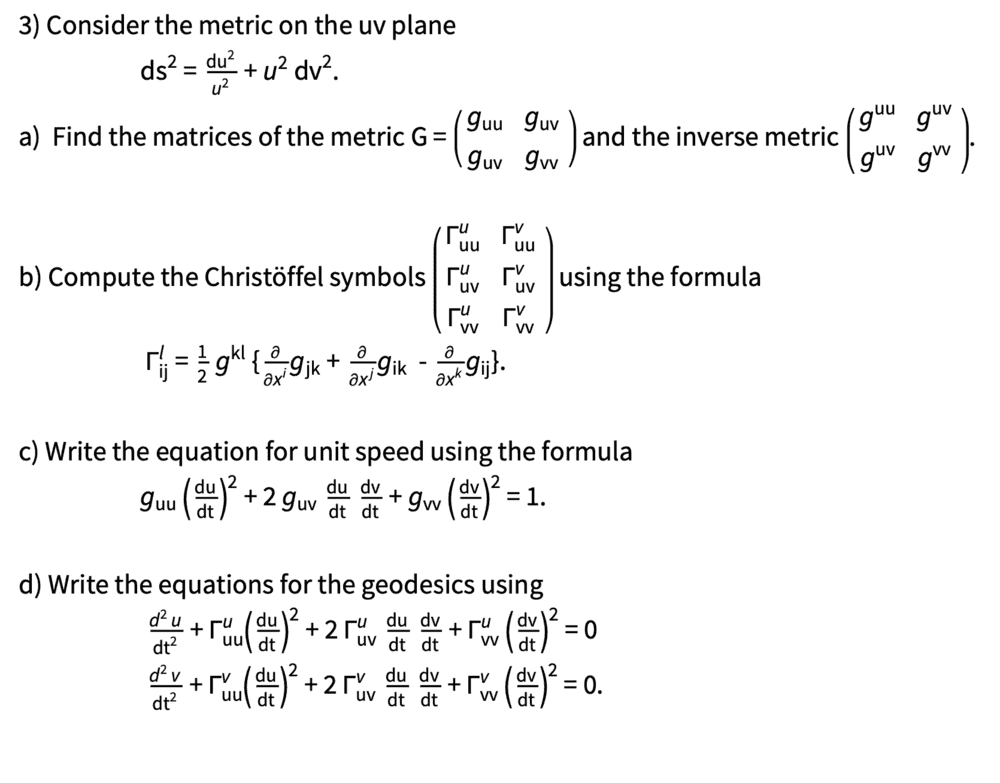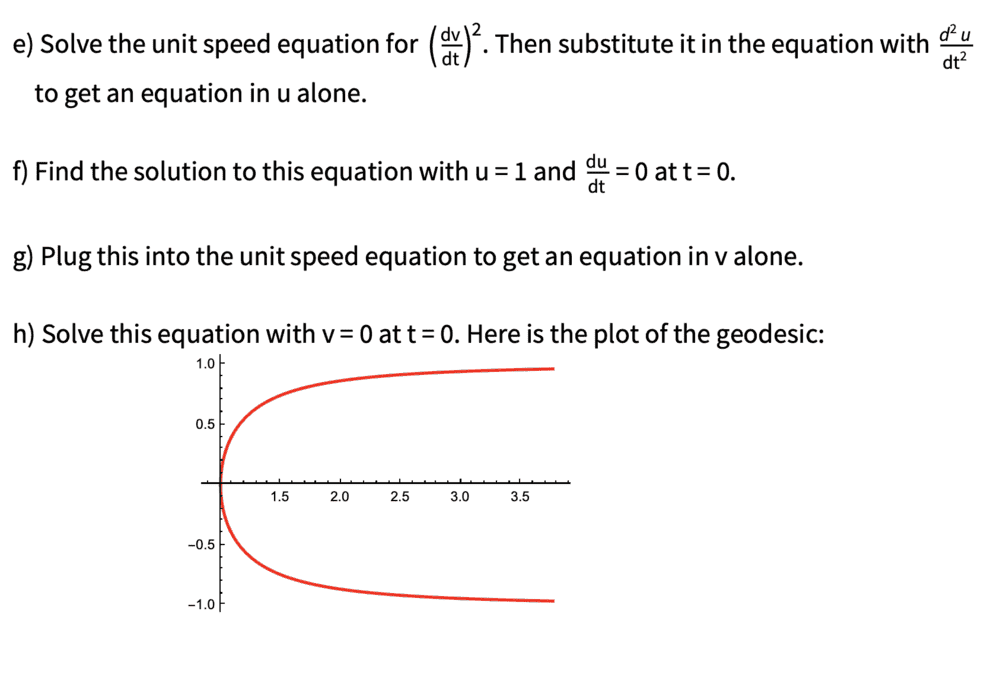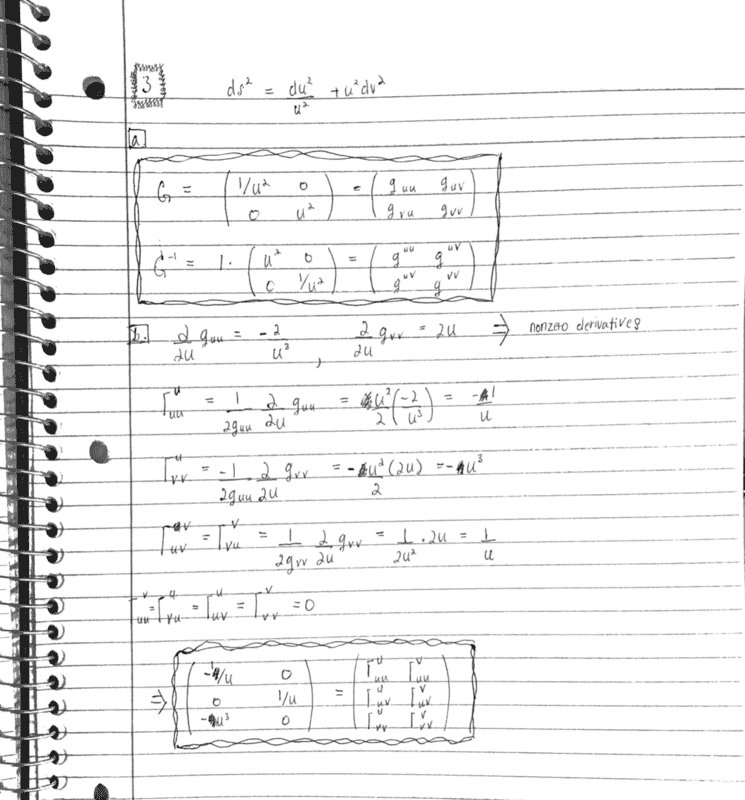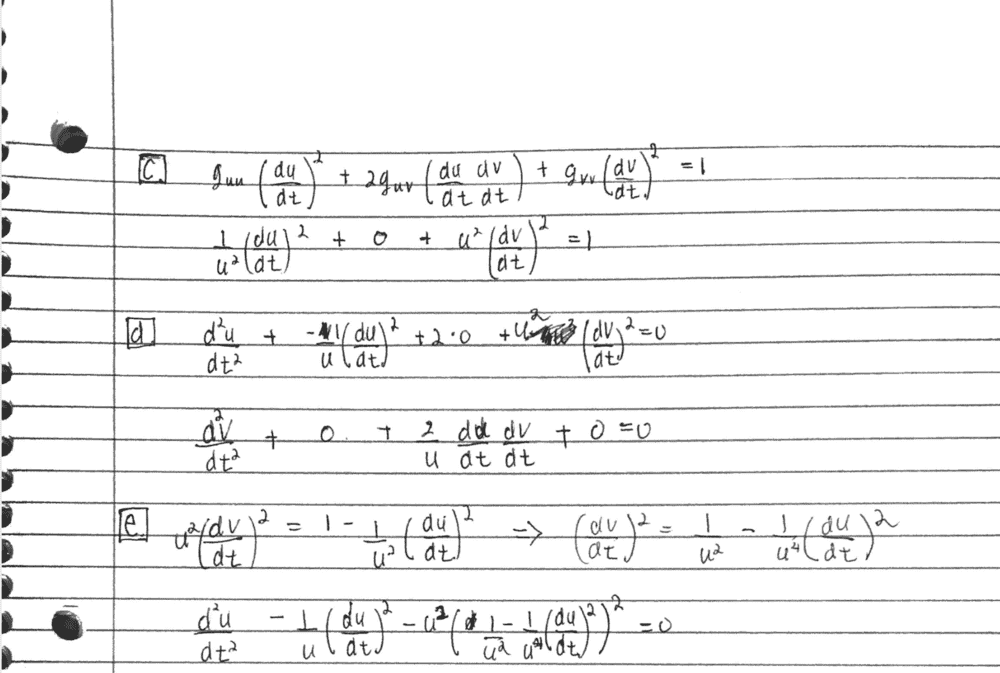I believe the last line is the correct ODE to solve for, which I was unable to solve using any methods I was familiar with. Looking at the plot of the geodesic, it looks like the V component asymptotically approaches 1 and -1 at infinite and -infinite t. the U component only exists for > 0 and is also symmetric around t = 0. Does anyone know of any similar ODE examples to learn from?

Last edited:
•Delta2
I think the best way to proceed is to use the hint from @aheight in post #6, and replace u'' with u' du'/du. This at least converts the equation to a first order equation.

•docnet
phyzguy said:
I think the best way to proceed is to use the hint from @aheight in post #6, and replace u'' with u' du'/du. This at least converts the equation to a first order equation.
... and beyond that, u'=u cos(θ) looks useful.

•docnet
haruspex said:
... and beyond that, u'=u cos(θ) looks useful.

Hm... I tried solving the expression u' = u cos(Θ) and obtained u = C exp [sin(Θ) ] which doesn't seem to correspond to a solution for our problem.

Last edited:
docnet said:
Hm... I tried solving the expression u' = u cos(Θ) and obtained u = C exp [sin(Θ) ] which doesn't seem to correspond to a solution for our problem.
No, I mean after replacing u'' with u' du'/du, substitute for u' using that relationship to get an ODE in x and θ.

•docnet
After doing the substitutions we ended up with the following equation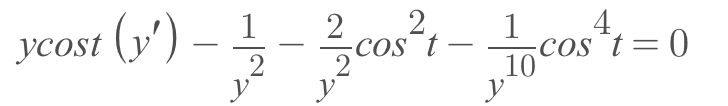where t = θ and u = y.

The 1st order ODE could not be solved by using Symbolab. Is there a mistake??

Last edited:
docnet said:
So we substitute u' = ucosΘ

and substitute u = (1/2)u2cosΘ obtained by integrating u'

and substitute du'/du = cosΘ obtained by differentiating u'

and ended up with the following oth order equation that cannot be solved for u.

https://www.physicsforums.com/attachments/274852

Should we substitute u' = ucosΘ and leave u and du'/du alone?

edit: the expression u = (1/2)u2cosΘ does not make any sense. I'll spend some time before posting a followup.
You have ##\ddot u=\frac{\dot u^2}u+\frac 1{u^2}(1-\frac{\dot u}u)^2##.
Writing ##x=u, y=\dot u, y'=\frac{dy}{dx}, \ddot u= \frac d{dt}y=\frac d{du}y\frac{du}{dt}=yy'##:
##x^2yy'=xy^2+(1-\frac{y^2}{x^2})^2##
If all that looks right to you, substitute ##y=x\cos(\theta)## and see what simplifies.

•docnet
haruspex said:
You have ##\ddot u=\frac{\dot u^2}u+\frac 1{u^2}(1-\frac{\dot u}u)^2##.
Writing ##x=u, y=\dot u, y'=\frac{dy}{dx}, \ddot u= \frac d{dt}y=\frac d{du}y\frac{du}{dt}=yy'##:
##x^2yy'=xy^2+(1-\frac{y^2}{x^2})^2##
If all that looks right to you, substitute ##y=x\cos(\theta)## and see what simplifies.

So we have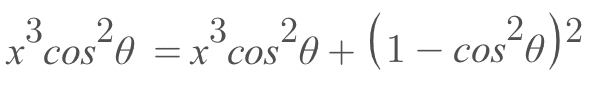which simplifies to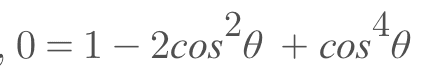then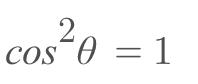which gives y = x in our substitution

and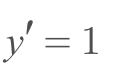with the solution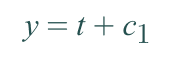and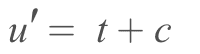with the solution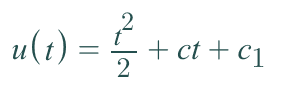with c1= 1 and c = 0.

May I continue solving the next part of the problem?

•docnet
haruspex said:
No, θ is a variable, so what does y' become?

Differentiating y with respect to x gives us y'=cosθ which gives

View attachment 274854

The first two terms cancel and we are left with the equation in θ as before.

when we differentiate y with respect to theta we have y' = - xsinθ which gives us an equation which is difficult to solve for x or θ.

We could differentiate with respect to x and theta using the chain rule, and it results in another equation which I was unable to solve for x or θ.

Last edited:
•Delta2
docnet said:
Differentiating y with respect to x gives us y'=cosθ which gives
No it doesn’t. θ is a variable, as in θ=θ(x).

•docnet
I'm getting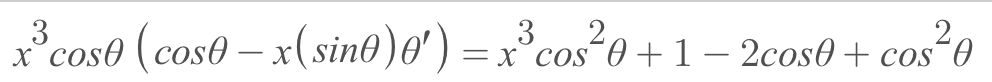which simplies to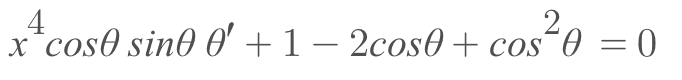whose solution is Θ(x) = 0

May I move onto the next part?

Last edited:
docnet said:
You have made a mistake in expanding ##(1-\frac{y^2}{x^2})^2##.

•docnet
haruspex said:
You have made a mistake in expanding (1−y2x2)2.

Doh! yes I did, and I just fixed my mistake.

It finally comes out to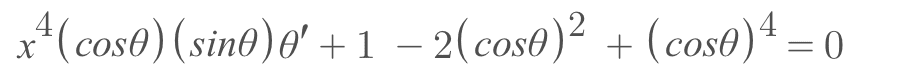whose solutions are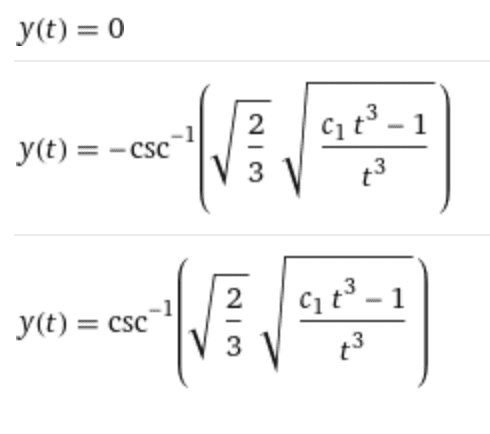where y(t) has been replaced with Θ(x) and t with x.

docnet said:
Doh! yes I did, and I just fixed my mistake.

It finally comes out to

View attachment 274923
Which can be simplified to ##x^4\sin(\theta)\cos(\theta)+\sin^4(\theta)=0##, hence ##x^4\cos(\theta)+\sin^3(\theta)=0##, unless sin() is 0.

•docnet
docnet said:
whose solutions are

View attachment 274922

where y(t) has been replaced with Θ(x) and t with x.
Defining a different y from what we had earlier is confusing.
At t=0, u=x=1, ##y=\dot u=0##, ##\cos(\theta)=0, |\csc(\theta)|=1##.

I get ##(\dot u)^2=\frac{2u^5-2u^2}{5u^3-2}##, leading to a nasty-looking integral.

Last edited:
•docnet
Is anyone familiar with the surface corresponding to our metric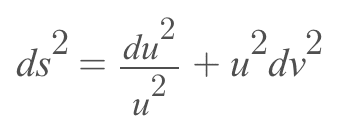?

It could give us an idea of what the geodesic is.

It looks like the surface stretches the du vector to infinity at u = 0 and shortens the dv vector to 0 at u = 0.

Dear all,

There is another error in our differential equation.

Starting over from part e) we solve the following equation for (dv/dt)2.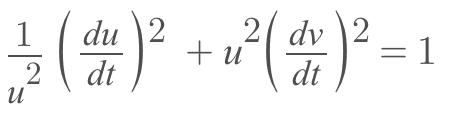giving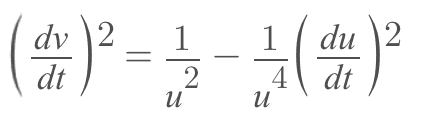and substitute it in the following equation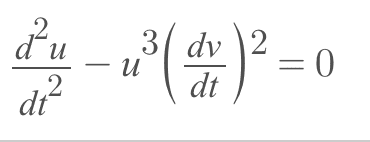giving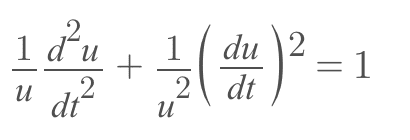Which offers no clear way to solve. We examine the following expression from part d).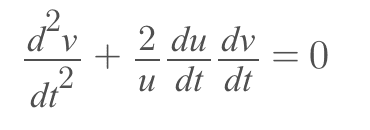which makes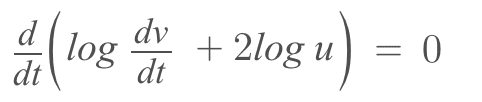which implies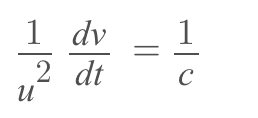for some constant c. solving,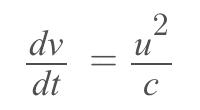substitute into the first equation to get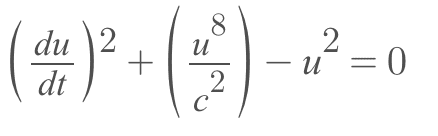which makes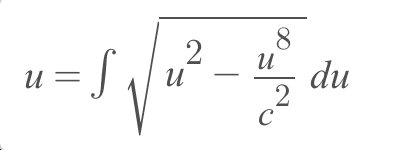I think this way is not easy.

Last edited:
The expression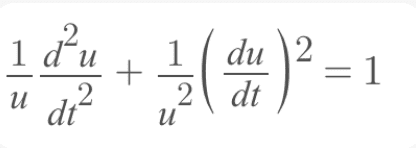has the solution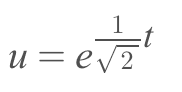and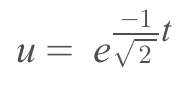For the v component of the geodesic, we plug in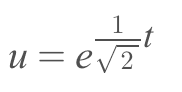into the unit speed equation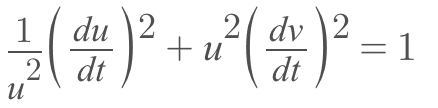giving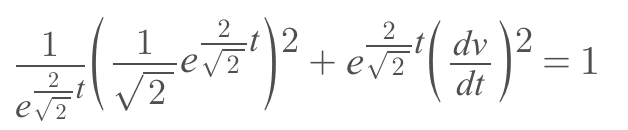and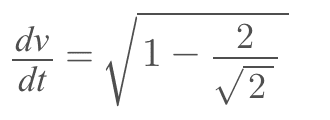We then integrate to give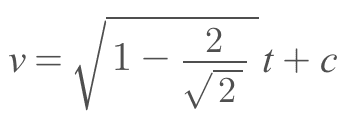and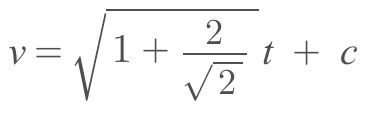which is incorrect judging from the following plot in the uv plane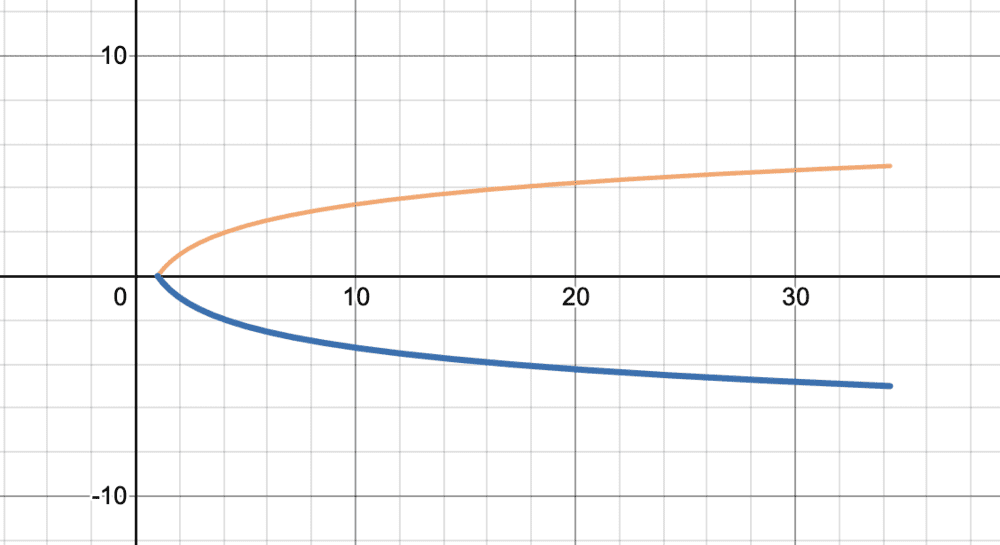but I think we are getting closer to the correct solution.

Last edited:
•docnet
What course is this? Differential geometry?

haruspex said:
You seem to have squared part of ##\frac{du}{dt}## twice. The left hand term above should reduce to 1/2.

I am sorry I should apologize because the solution to our ODE is not a geodesic, because we are solving the wrong ODE.

Not solving this problem has been eating me up. So, I revisited our previous calculations tonight, made sure to be extra careful not to make errors and ended up with the following. I still have not learned how to write LaTex equations, so these were typed in Microsoft Word, and these will not show up in quoted messages.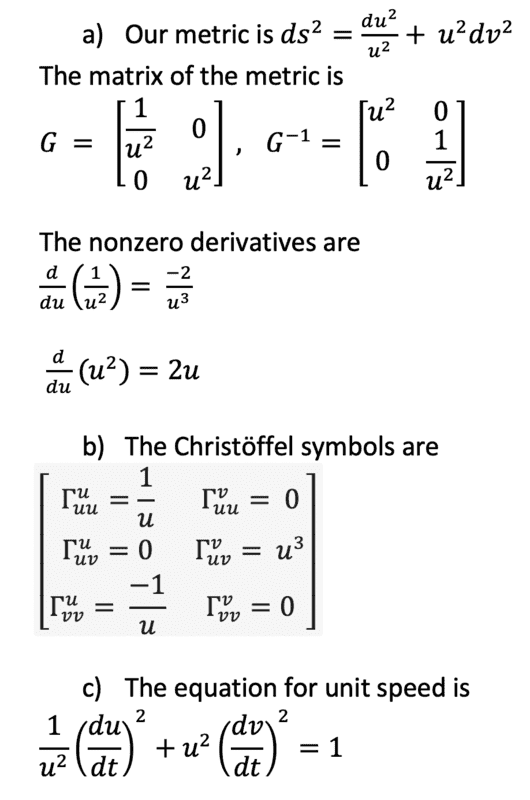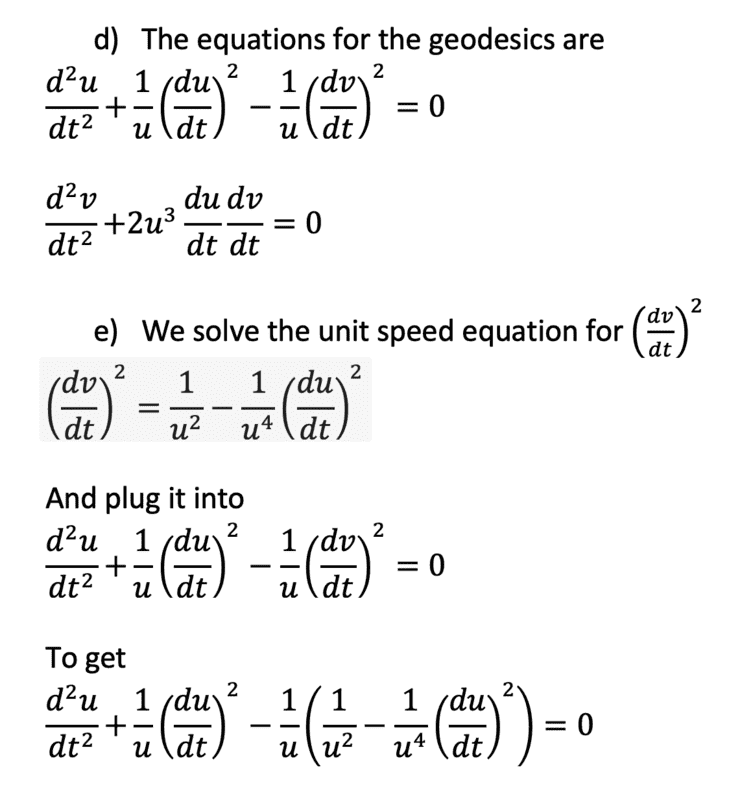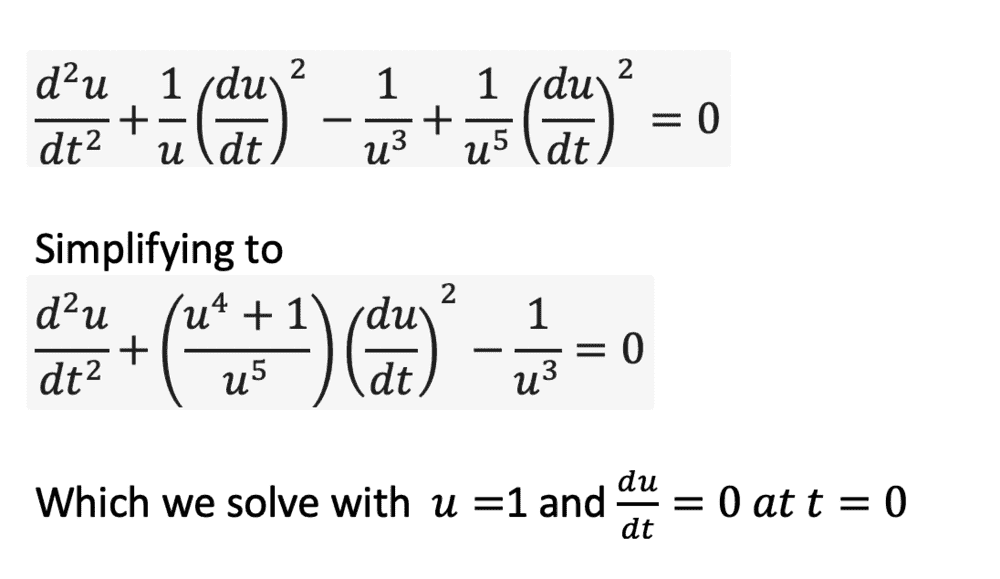I am unable to solve this nonlinear second order ODE by any method I am familiar with.

Is anyone able to compute it using Mathematica or Wolfram Αlpha?

Thank you.

Last edited:
Acccording to DSolve in Mathematica, the fearful ODE $$\frac{d^2u}{dt^2}=\frac{1}{u^3}-\Big(\frac{u^4+1}{u^5}\Big)\Big(\frac{du}{dt}\Big)^2$$
has "closed form" solutions given by...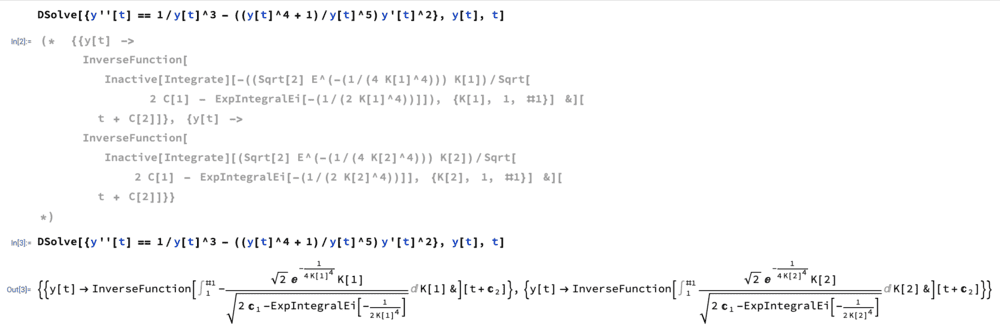By using DSolve with initial value conditions,

$$\frac{d^2u}{dt^2}=\frac{1}{u^3}-\Big(\frac{u^4+1}{u^5}\Big)\Big(\frac{du}{dt}\Big)^2\Rightarrow u(t)=\sqrt{1+t^2}$$

(g) we plug ##u(t)## into the unit speed equation to find
$$\frac{dv}{dt}=\Big(\frac{t^4+t^2+1}{(1+t^2)^3}\Big)^{\frac{1}{2}}$$

which is a separable equation, when trying to compute
$$v(t)=\int \Big(\frac{t^4+t^2+1}{(1+t^2)^3}\Big)^{\frac{1}{2}}dt$$

we cannot find an explicit closed form solution